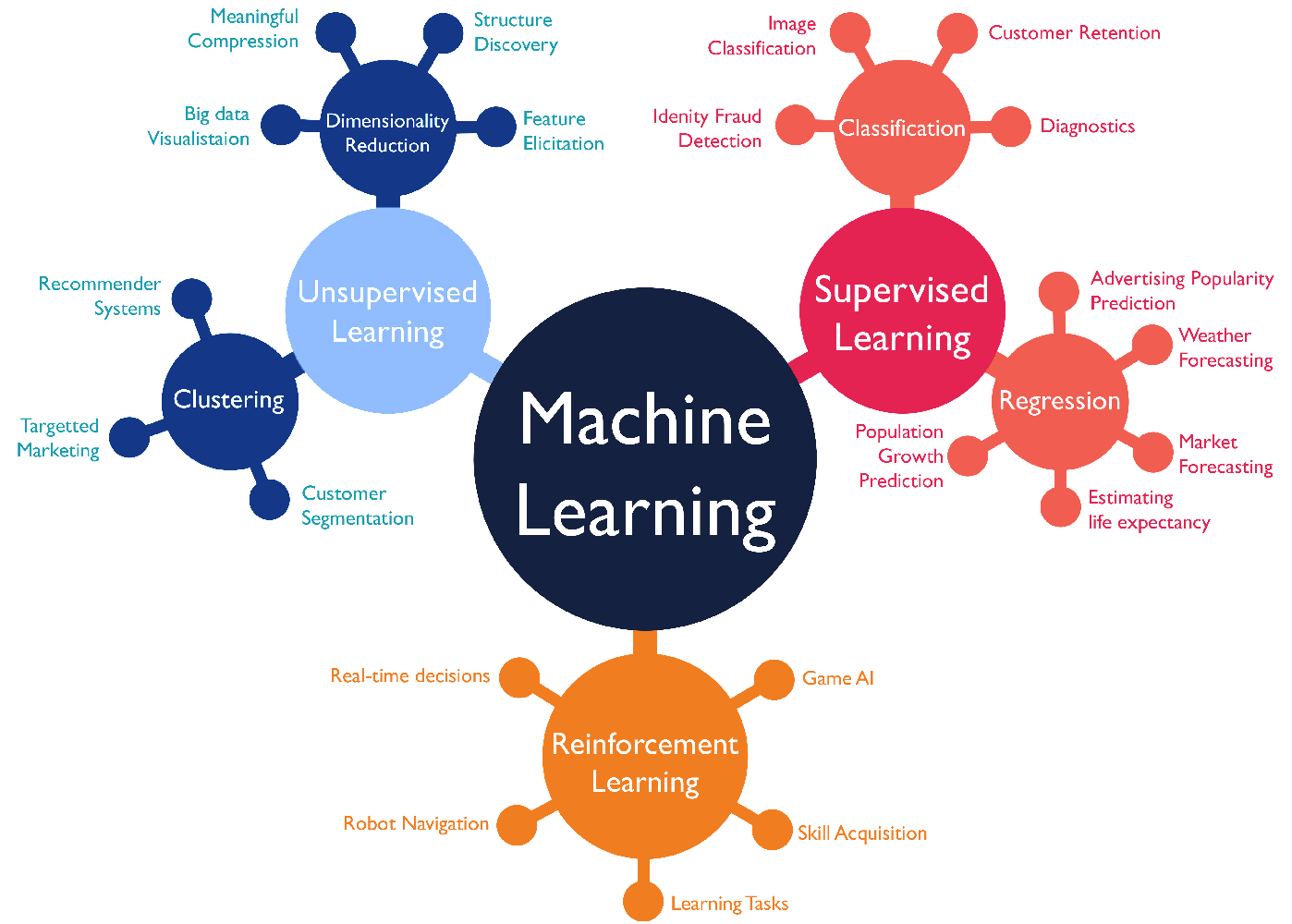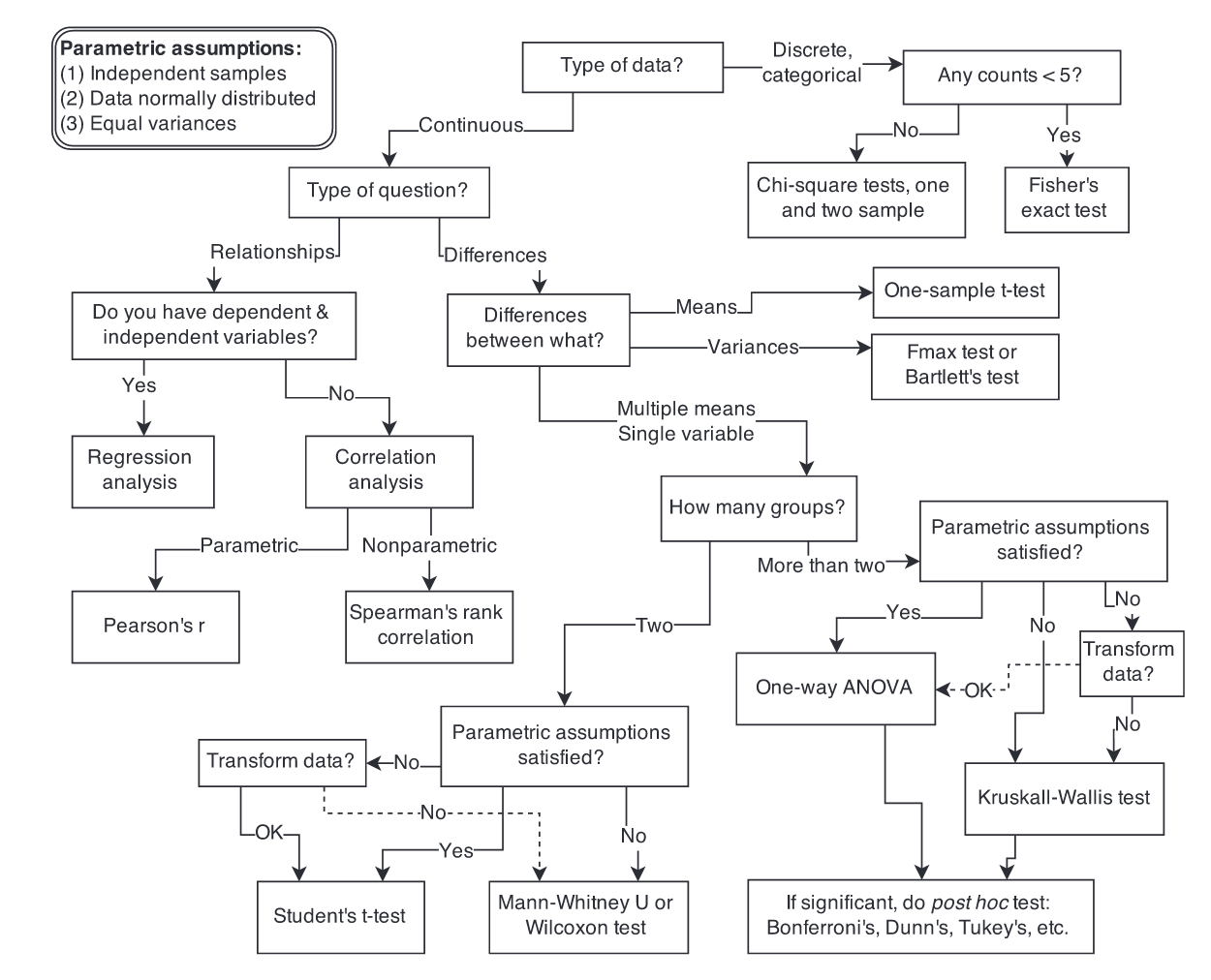# Machine Learning NotesML breakdown: Supervised + Unsupervised + RL
• Expectation-maximization (EM): assumes random components and computes for each point a probability of being generated by each component of the model. Then iteratively tweaks the parameters to maximize the likelihood of the data given those assignments. Example: Gaussian Mixture
• Gradient Boosting: optimization of arbitrary differentiable loss functions. — Risk of overfitting
• Hypothesis testsSelecting statistical test. Source: Statistical Rethinking 2. Free Chapter 1
• KNN: + Simple, flexible, naturally handles multiple classes. — Slow at scale, sensitive to feature scaling and irrelevant features
• K-means: aims to choose centroids that minimize the inertia, or within-cluster sum-of-squares criterion. Use the “elbow” method to identify the right number of means
• Lasso: linear model regularization technique with tendency to prefer solutions with fewer non-zero coefficients
• Linear Discriminant Analysis (LDA): A classifier with a linear decision boundary, generated by fitting class conditional densities to the data and using Bayes’ rule. The model fits a Gaussian density to each class, assuming that all classes share the same covariance matrix.
• Linear regression assumptions (LINE): 1) Linearity, 2) Independence of errors, 3) Normality of errors, 4) Equal variances. Tests of assumptions: i) plot each feature on x-axis vs y_error, ii) plot y_predicted on x-axis vs y_error, iii) histogram of errors
• Overfitting, bias-variance and learning curves. Overfitting (high variance) options: more data, increase regularization, or decrease model complexity
• Overspecified model: can be used for prediction of the label, but should not be used to ascribe the effect of a feature on the label
• PCA: transform data using k vectors that minimize the perpendicular distance to points. PCA can be also thought of as an eigenvalue/engenvector decomposition
• Receiver operating characteristic (ROC): relates true positive rate (y-axis) and false positive rate (x-axis). A confusion matrix defines TPR = TP / (TP + FN) and FPR = FP / (FP + TN)
• Preprocessing: duplicates -> outliers -> missing values -> feature correlation -> feature distribution/skew
• Naive Bayes
• Normal Equation
• Random Forests: each tree is built using a sample of rows (with replacement) from training set. + Less prone to overfitting
• Reinforcement Learning